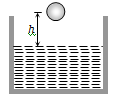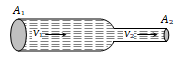Spherical balls of radius 'r' are falling in a viscous fluid of viscosity '$\mathrm{\eta }$' with a velocity 'v'. The retarding viscous force acting on the spherical ball is

(a) Inversely proportional to 'r' but directly proportional to velocity 'v'

(b) Directly proportional to both radius 'r' and velocity 'v'

(c) Inversely proportional to both radius 'r' and velocity 'v'

(d) Directly proportional to 'r' but inversely proportional to 'v'

Concept Questions :-

Viscosity
High Yielding Test Series + Question Bank - NEET 2020

Difficulty Level:

A small sphere of mass m is dropped from a great height. After it has fallen 100 m, it has attained its terminal velocity and continues to fall at that speed. The work done by air friction against the sphere during the first 100 m of fall is

(a) Greater than the work done by air friction in the second 100 m

(b) Less than the work done by air friction in the second 100 m

(c) Equal to 100 mg

(d) Greater than 100 mg

Concept Questions :-

Viscosity
High Yielding Test Series + Question Bank - NEET 2020

Difficulty Level:

Two drops of the same radius are falling through air with a steady velocity of 5 cm per sec. If the two drops coalesce, the terminal velocity would be

(a) 10 cm per sec                                  (b) 2.5 cm per sec
(c) $5×\left(4{\right)}^{1}{3}}$ cm per sec                          (d) $5×\sqrt{2}$ cm per sec

Concept Questions :-

Surface tension
High Yielding Test Series + Question Bank - NEET 2020

Difficulty Level:

A ball of radius r and density $\mathrm{\rho }$ falls freely under gravity through a distance h before entering water. Velocity of ball does not change even on entering water. If viscosity of water is $\mathrm{\eta }$, the value of h is given by(a) $\frac{2}{9}{\mathrm{r}}^{2}\left(\frac{1-\mathrm{\rho }}{\mathrm{\eta }}\right)\mathrm{g}$

(b) $\frac{2}{81}{\mathrm{r}}^{2}\left(\frac{\mathrm{\rho }-1}{\mathrm{\eta }}\right)\mathrm{g}$

(c) $\frac{2}{81}{\mathrm{r}}^{4}{\left(\frac{\mathrm{\rho }-1}{\mathrm{\eta }}\right)}^{2}\mathrm{g}$

(d) $\frac{2}{9}{\mathrm{r}}^{4}{\left(\frac{\mathrm{\rho }-1}{\mathrm{\eta }}\right)}^{2}\mathrm{g}$

Concept Questions :-

Viscosity
High Yielding Test Series + Question Bank - NEET 2020

Difficulty Level:

The rate of steady volume flow of water through a capillary tube of length 'l' and radius 'r' under a pressure difference of P is V. This tube is connected with another tube of the same length but half the radius in series. Then the rate of steady volume flow through them is (The pressure difference across the combination is P)

(a) $\frac{\mathrm{V}}{16}$                                     (b) $\frac{\mathrm{V}}{17}$

(c) $\frac{16\mathrm{V}}{17}$                                    (d) $\frac{17\mathrm{V}}{16}$

Concept Questions :-

Viscosity
High Yielding Test Series + Question Bank - NEET 2020

Difficulty Level:

A liquid is flowing in a horizontal uniform capillary tube under a constant pressure difference P. The value of pressure for which the rate of flow of the liquid is doubled when the radius and length both are doubled is

(a) P                             (b) $\frac{3\mathrm{P}}{4}$

(c) $\frac{\mathrm{P}}{2}$                           (d) $\frac{\mathrm{P}}{4}$

Concept Questions :-

Viscosity
High Yielding Test Series + Question Bank - NEET 2020

Difficulty Level:

We have two (narrow) capillary tubes T1 and T2. Their lengths are l1 and l2 and radii of cross-section are r1 and r2 respectively. The rate of flow of water under a pressure difference P through tube T1 is 8cm3/sec. If l1 = 2l2 and r1 =r2, what will be the rate of flow when the two tubes are connected in series and pressure difference across the combination is same as before (= P)

(a) 4 cm3/sec                           (b) (16/3) cm3/sec

(c) (8/17) cm3/sec                    (d) None of these

Concept Questions :-

Viscosity
High Yielding Test Series + Question Bank - NEET 2020

Difficulty Level:

The Reynolds number of a flow is the ratio of

(a) Gravity to viscous force

(b) Gravity force to pressure force

(c) Inertia forces to viscous force

(d) Viscous forces to pressure forces

Concept Questions :-

Types of flow

Difficulty Level:

Water is flowing through a tube of non-uniform cross-section ratio of the radius at entry and exit end of the pipe is 3 : 2. Then the ratio of velocities at entry and exit of liquid is -

(a) 4 : 9                          (b) 9 : 4
(c) 8 : 27                         (d) 1 : 1

Concept Questions :-

Equation of continuity
High Yielding Test Series + Question Bank - NEET 2020

Difficulty Level:

A liquid flows in a tube from left to right as shown in figure. ${\mathrm{A}}_{1}$ and ${\mathrm{A}}_{2}$ are the cross-sections of the portions of the tube as shown. Then the ratio of speeds ${\mathrm{v}}_{1}/{\mathrm{v}}_{2}$ will be(a) ${\mathrm{A}}_{1}/{\mathrm{A}}_{2}$

(b) ${\mathrm{A}}_{2}/{\mathrm{A}}_{1}$

(c) $\sqrt{{\mathrm{A}}_{2}}/\sqrt{{\mathrm{A}}_{1}}$

(d) $\sqrt{{\mathrm{A}}_{1}}/\sqrt{{\mathrm{A}}_{2}}$

Concept Questions :-

Equation of continuity